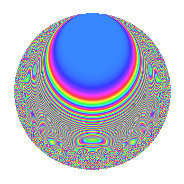# Properties

 Label 8015.2.a.mLevel 8015 Weight 2 Character orbit 8015.a Self dual Yes Analytic conductor 64.000 Analytic rank 0 Dimension 67 CM No

# Related objects

## Newspace parameters

 Level: $$N$$ = $$8015 = 5 \cdot 7 \cdot 229$$ Weight: $$k$$ = $$2$$ Character orbit: $$[\chi]$$ = 8015.a (trivial)

## Newform invariants

 Self dual: Yes Analytic conductor: $$64.0000972201$$ Analytic rank: $$0$$ Dimension: $$67$$ Fricke sign: $$-1$$ Sato-Tate group: $\mathrm{SU}(2)$

## $q$-expansion

The dimension is sufficiently large that we do not compute an algebraic $$q$$-expansion, but we have computed the trace expansion.

 $$\operatorname{Tr}(f)(q) =$$ $$67q + 3q^{2} + 73q^{4} + 67q^{5} + 17q^{6} - 67q^{7} + 12q^{8} + 97q^{9} + O(q^{10})$$ $$\operatorname{Tr}(f)(q) =$$ $$67q + 3q^{2} + 73q^{4} + 67q^{5} + 17q^{6} - 67q^{7} + 12q^{8} + 97q^{9} + 3q^{10} + 11q^{11} + 9q^{12} + 19q^{13} - 3q^{14} + 93q^{16} + 7q^{17} + 9q^{18} + 36q^{19} + 73q^{20} - 8q^{22} - 2q^{23} + 37q^{24} + 67q^{25} + 39q^{26} + 6q^{27} - 73q^{28} + 56q^{29} + 17q^{30} + 63q^{31} + 27q^{32} + 51q^{33} + 55q^{34} - 67q^{35} + 148q^{36} + 28q^{37} + 10q^{38} + 7q^{39} + 12q^{40} + 84q^{41} - 17q^{42} - 11q^{43} + 41q^{44} + 97q^{45} + 17q^{46} - 10q^{47} + 10q^{48} + 67q^{49} + 3q^{50} - q^{51} + 49q^{52} + 3q^{53} + 20q^{54} + 11q^{55} - 12q^{56} + 45q^{57} + 22q^{58} + 80q^{59} + 9q^{60} + 64q^{61} - 37q^{62} - 97q^{63} + 110q^{64} + 19q^{65} + 75q^{66} + 22q^{67} + 7q^{68} + 107q^{69} - 3q^{70} + 24q^{71} + 72q^{72} + 83q^{73} + 52q^{74} + 115q^{76} - 11q^{77} - 70q^{78} - 32q^{79} + 93q^{80} + 183q^{81} + 56q^{82} - 58q^{83} - 9q^{84} + 7q^{85} + 51q^{86} + 20q^{87} - 5q^{88} + 129q^{89} + 9q^{90} - 19q^{91} - 37q^{92} + 33q^{93} + 89q^{94} + 36q^{95} + 129q^{96} + 126q^{97} + 3q^{98} - 27q^{99} + O(q^{100})$$

## Embeddings

For each embedding $$\iota_m$$ of the coefficient field, the values $$\iota_m(a_n)$$ are shown below.

For more information on an embedded modular form you can click on its label.

Label $$a_{2}$$ $$a_{3}$$ $$a_{4}$$ $$a_{5}$$ $$a_{6}$$ $$a_{7}$$ $$a_{8}$$ $$a_{9}$$ $$a_{10}$$
1.1 −2.79998 −2.08197 5.83989 1.00000 5.82947 −1.00000 −10.7516 1.33460 −2.79998
1.2 −2.72902 1.60790 5.44753 1.00000 −4.38799 −1.00000 −9.40835 −0.414653 −2.72902
1.3 −2.66598 2.60914 5.10744 1.00000 −6.95591 −1.00000 −8.28438 3.80761 −2.66598
1.4 −2.55168 −1.07267 4.51109 1.00000 2.73711 −1.00000 −6.40752 −1.84938 −2.55168
1.5 −2.54658 −2.85949 4.48508 1.00000 7.28192 −1.00000 −6.32845 5.17667 −2.54658
1.6 −2.53656 −2.78475 4.43415 1.00000 7.06368 −1.00000 −6.17438 4.75481 −2.53656
1.7 −2.40339 −1.71467 3.77630 1.00000 4.12102 −1.00000 −4.26915 −0.0599106 −2.40339
1.8 −2.30662 1.34001 3.32049 1.00000 −3.09089 −1.00000 −3.04587 −1.20437 −2.30662
1.9 −2.25277 3.29965 3.07495 1.00000 −7.43334 −1.00000 −2.42162 7.88769 −2.25277
1.10 −2.05677 1.58909 2.23031 1.00000 −3.26839 −1.00000 −0.473688 −0.474802 −2.05677
1.11 −1.94503 2.18285 1.78313 1.00000 −4.24571 −1.00000 0.421811 1.76484 −1.94503
1.12 −1.89706 −1.32001 1.59885 1.00000 2.50414 −1.00000 0.761013 −1.25758 −1.89706
1.13 −1.83785 1.36680 1.37770 1.00000 −2.51199 −1.00000 1.14369 −1.13184 −1.83785
1.14 −1.82956 −1.24341 1.34731 1.00000 2.27489 −1.00000 1.19414 −1.45394 −1.82956
1.15 −1.80341 0.567965 1.25230 1.00000 −1.02428 −1.00000 1.34841 −2.67742 −1.80341
1.16 −1.76232 −3.28691 1.10578 1.00000 5.79259 −1.00000 1.57590 7.80377 −1.76232
1.17 −1.76209 −0.304110 1.10496 1.00000 0.535869 −1.00000 1.57714 −2.90752 −1.76209
1.18 −1.50021 0.266806 0.250644 1.00000 −0.400267 −1.00000 2.62441 −2.92881 −1.50021
1.19 −1.44039 2.88342 0.0747197 1.00000 −4.15325 −1.00000 2.77315 5.31413 −1.44039
1.20 −1.42994 −3.33526 0.0447232 1.00000 4.76921 −1.00000 2.79592 8.12394 −1.42994
See all 67 embeddings
 $$n$$: e.g. 2-40 or 990-1000 Embeddings: e.g. 1-3 or 1.67 Significant digits: Format: Complex embeddings Normalized embeddings Satake parameters Satake angles

## Inner twists

This newform does not have CM; other inner twists have not been computed.

## Atkin-Lehner signs

$$p$$ Sign
$$5$$ $$-1$$
$$7$$ $$1$$
$$229$$ $$1$$

## Hecke kernels

This newform can be constructed as the intersection of the kernels of the following linear operators acting on $$S_{2}^{\mathrm{new}}(\Gamma_0(8015))$$:

 $$T_{2}^{67} - \cdots$$ $$T_{3}^{67} - \cdots$$